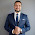## Friday, January 7, 2011

### ActionScript 3: Convert Strings to Numbers

In this lesson, we are going to learn how to convert strings to numbers in AS3.

You can convert strings that are made up of numerical characters into actual Number data using the Number() constructor. The way it works is that you pass the String value to the Number() constructor, and in turn, this will create a Number version of the String that was passed to it.

Example:
``````var myString:String = "7";
var myNumber = Number(myString);``````
The first line creates a string - myString with a value of 7. The second line takes that String data and passes it to the Number() constructor, which creates a number version from it. And the converted value, which is now of the Number data type, is assigned to the variable named myNumber.

The syntax would be:
Number(String)

Why would I want to convert strings to numbers in AS3?
Let's say you have some String data made up of numerical characters, and you'd like to perform arithmetic operations using that data. If you don't convert the strings to numbers, then Flash won't allow you to perform arithmetic operations on them.

Here's an example. Let's say we have 2 strings that are made up of numerical characters:
``````var s1:String = "2";
var s2:String = "5";``````
And then we try to multiply them:
`trace(s1 * s2);`
Flash is going to give us this error message:
1067: Implicit coercion of a value of type String to an unrelated type Number.
This error is telling us that we are trying to force (or coerce) the String data to be used as Number data. You can only multiply numbers, NOT strings. So in the act of trying to multiply String data, we are implicitly coercing the values to change into Number data. We can't do that. We should explicitly convert the String data to Number data first, and then perform the arithmetic operation.

Here's another example. If we use the + operator on strings, then the strings will simply be joined together instead of the values being added to create a sum. Let's take a look:
``````var s1:String = "2";
var s2:String = "5";
trace(s1 + s2);``````
Here, we have two strings - s1 = "2" and s2 = "5". If we were to use the + operator on these two values, the result would be 25. The values of s1 (2) and s2 (5) will simply be joined together in the same way that letters are joined together in order to make words. If these were actual Number data, then the result of adding 2 and 5 would be 7 instead.

So if we want the values to be added to create a sum, then we'll need to use the Number() constructor to create number versions out of the strings:
``````var s1:String = "2";
var s2:String = "5";
trace( Number(s1) + Number(s2) );``````
Here, the strings s1 and s2 are each passed to the Number() constructor. This will create number versions from those strings, which can then be used in arithmetic operations. So here, the result will be 7, instead of the 25 we got from the previous example.

Here's another similar example. If you'd like to use the contents of a TextField instance in arithmetic operations, then you will also need to use the Number() constructor to create number values from those numerical characters inside the text field. Remember that characters inside a TextField are of the String data type, which is why we'll need to convert them to the Number data type for usage in arithmetic operations. For example:
``````// Assume that tf1 and tf2 are instances of the TextField class
// and that they have already been created and added to the stage
tf1.text = "2";
tf2.text = "5";
trace( Number(tf1.text) + Number(tf2.text) );``````
So here, in the last line, we are retrieving the values inside the text fields (tf1 contains "2", tf2 contains "5"). Those values are passed to the Number() constructor which will create number versions from those values. Those numbers can then be used in the arithmetic operation. The result in this example would be 7.

And that is how you can convert strings to numbers in ActionScript 3.

1.hello. I´ve got a question. what should I do if I´ve got Two textInput instances (input 1 & input2) and a third instance (output) and I want to type numbers in "input1" and "input2" and get the sum of them in "output" without having to use a Mouse event. In other words, I want an autosum.
I have this cide:

import fl.managers.StyleManager;
this.stop();
var Format1:TextFormat = new TextFormat();
Format1.size = 17;
Format1.font = "Arial Rounded MT Bold";
Format1.align = "right";

StyleManager.setComponentStyle(TextInput, "textFormat", Format1);

output.text = String(Number(input1.text) + Number(input2.text));

1.I used the keyboard event to resolve this issue, in two ways, TAB and ENTER

import flash.text.TextField;
import flash.text.TextInteractionMode;
import flash.ui.Keyboard;
import flash.events.KeyboardEvent;
import flash.events.Event;

var Label1: TextField = new TextField();
var Label2: TextField = new TextField();
var Result: TextField = new TextField();
var Input1: TextField = new TextField();
var Input2: TextField = new TextField();

//Label1
Label1.width = 20;
Label1.x = 75;
Label1.y = 100;
Label1.text = "v1";

//value 1
Input1.border = true;
Input1.width = 30;
Input1.height = 20;
Input1.x = 100;
Input1.y = 100;
Input1.type = "input";
Input1.restrict = "0-9";
Input1.maxChars = 3;

//Label 2
Label2.width = 30;
Label2.x = 130;
Label2.y = 100;
Label2.text = "+ v2";

//value 2
Input2.border = true;
Input2.width = 30;
Input2.height = 20;
Input2.x = 170;
Input2.y = 100;
Input2.type = "input";
Input2.restrict = "0-9";
Input2.maxChars = 3;

//Label Result
Result.width = 50;
Result.height = 30;
Result.x = 210;
Result.y = 100;
Result.text = " = " + String(Number(Input1.text) + Number(Input2.text));

stage.focus = Input1;

function onKeyPressed(e: KeyboardEvent): void {
if (e.keyCode == Keyboard.ENTER) {
Result.text = " = " + String(Number(Input1.text) + Number(Input2.text));
}
}

function onTabMove(e: KeyboardEvent): void {
if (e.keyCode == Keyboard.TAB) {
Result.text = " = " + String(Number(Input1.text) + Number(Input2.text));
}
}